Next: Quantum line cut Up: Simulation of STM images Previous: Experiment

# Geometric line cut

As a first approximation, we have calculated the image distortions caused by finite tip size using a simple geometric method (''geometric line cut'').

Model system is shown on Fig. 3.. Effective surface of electrodes (Cf. Fig. 4.) is assumed to be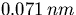outside the geometric surface.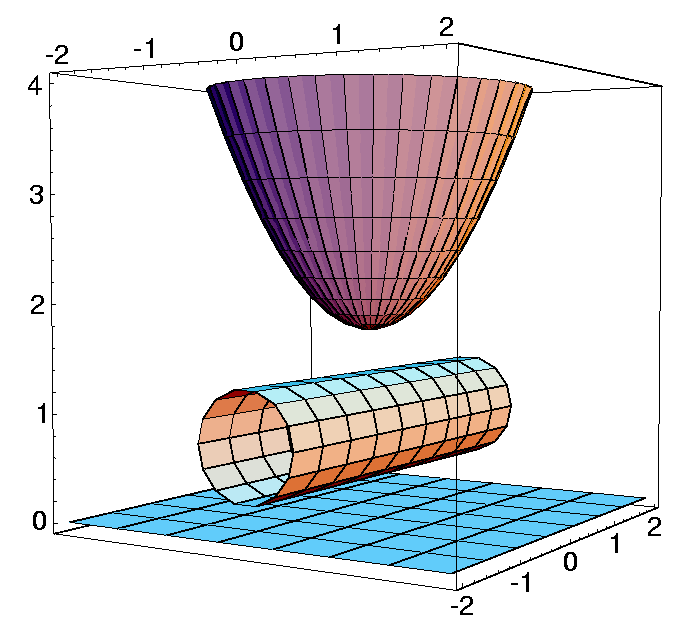Figure 3: Model system. Geometric surface of the support, tube, and tip.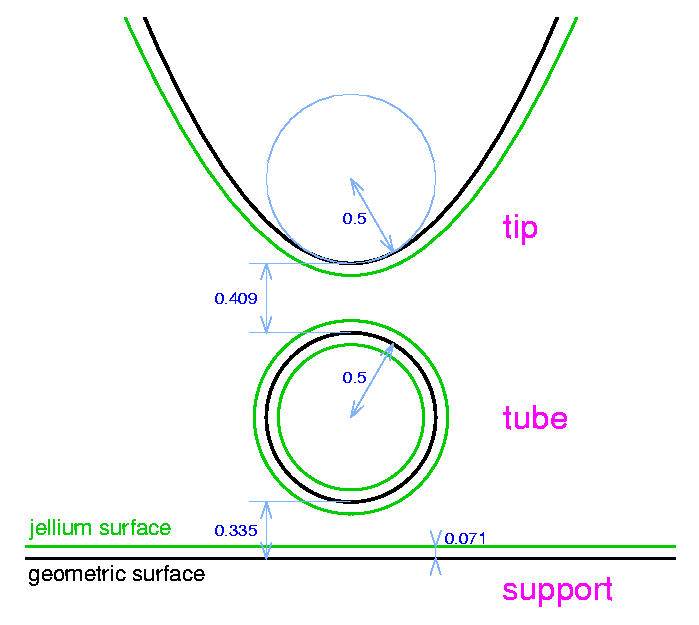Figure 4: Comparison of effective and geometric surfaces of electrodes. Effective surface (green line) isoutside of the geometric surface (black line). Dimensions are in nm.

Geometric line cut is shown in Fig. 5.. It is defined as the line drawn by the tip apex point ( (Xapex, Zapex), when the distance of the nearest point of the sample and tip is the (pre-selected) constant value. When the tip is far from the nanotube the line cut is a horizontal line above the support surface. In this case the nearest point of the tip to the sample is the tip apex point. When the tip approaches the nanotube (at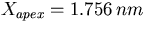) the nearest tip point to the sample becomes a point on the side of the tip and the nearest sample point to the tip will be a point on the side of the nanotube.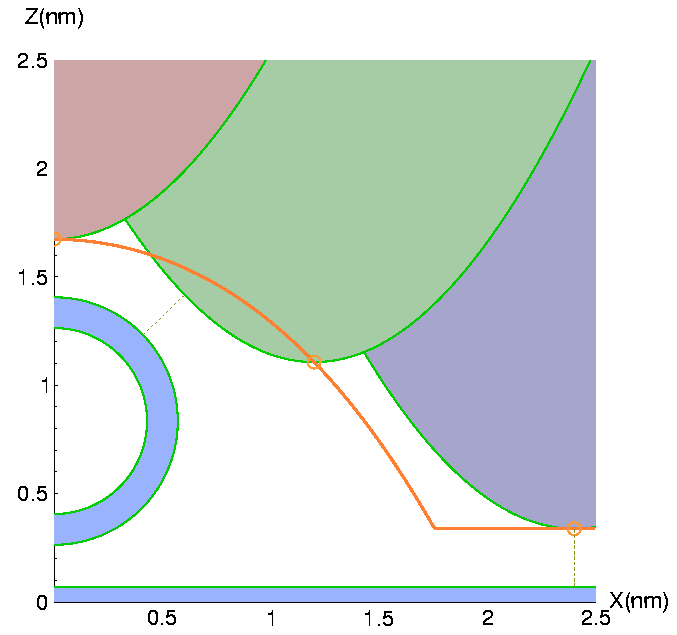Figure 5: Geometric line cut. Red line is the geometric line cut drawn by the tip apex (marked by open circle). Shaded lower half plane, middle ring and upper hyperbolas show the vertical cross sections of sample, nanotube, and tip. Electrodes are bounded by their jellium surfaces (green lines). Several, typical tip positions are shown, with different colors. For each tip position, nearest tip and sample points are joined by a thin dashed line.Next: Quantum line cut Up: Simulation of STM images Previous: Experiment 